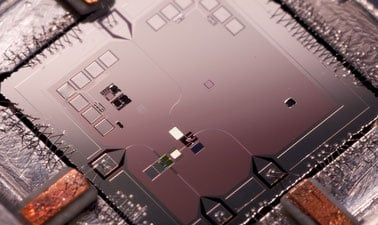Introduction to Quantum Computing for Everyone

This first course in quantum computing is for novices and requires learners to have only basic algebra. It covers the future impacts of quantum computing, provides intuitive introductions of quantum physics phenomenon, and progresses from single operations to a complete algorithm.There is one session available:

5,173 already enrolled! After a course session ends, it will be archivedOpens in a new tab.
Starts Jan 14
Ends Jul 31
Estimated 5 weeks
3–5 hours per week
Self-paced
Free

Quantum computing is coming closer to reality, with 80+ bit machines in active use. This course provides an intuitive introduction to the impacts, underlying phenomenon, and programming principles that underlie quantum computing.

The course begins with an exploration of classes of computational problems that classical computers are not well-suited to solve. We then progress to an intuitive introduction to key QIS concepts that underlie quantum computing. Next, we introduce individual quantum operations, but with a symbolic representation and mathematical representation. A limited set of linear algebra operations will be taught so that students can calculate operation results. Finally, we string these individual operations together to create the first algorithm that illustrates the performance advantage resulting from these unique operations.

What you'll learn

Skip What you'll learn

● Which types of applications may benefit from quantum computing
● Quantum physics principles and how they affect quantum computing
● Mathematical representation of quantum state
● Individual quantum operations
● Mathematical operations to calculate quantum operations
● Representation of multi-operation sequences
● Deutsch’s algorithm

Syllabus

Skip Syllabus

QIS Applications & Hardware
Quantum Operations,
Qubit Representation
Measurement
Superposition
Matrix Multiplication
Multi-Qubit Operations
Quantum Circuits
Entanglement
Deutsch’s Algorithm